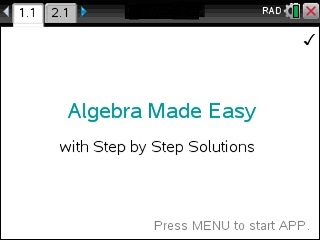# Step by Step Algebra app for the Ti-Nspire – Watch this Video at https://youtu.be/Q0uXzdzzHogStep by Step Algebra App for the Ti-NSpire at www.tinspireapps.com. It covers the ENTIRE Algebra curriculum: Algebra, Functions and their Analysis, Trig, Geometry, Complex Numbers, Matrices, Exponential/Logarithmic Functions, Probabilities, Combinatorics, Sequences, Induction, Sigma Notation, etc

• Step by Step – Complete the Square
• Step by Step – Partial Fractions
• Step by Step – Complex Numbers
• Step by Step – Powers
• Step by Step – Roots
• Step by Step – Synthetic Division
• Step by Step – Radicals
• Logarithm and Exponential Solver
• Rewrite Logarithms into their Exponential Form and back: log_b(y)=x <–> y=b^x
• Expand and Condense Logarithms Step by Step
• Rule of 72, Change of Logarithm base
• Effective Interest rates
• Euler Number as Limit Definition.
• Money Growth Solver